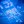# Three Transistor OscillatorCrazy Circuits wrote 03/21/2020 at 20:04 • 4 min read • Like

This article shows you how to make a simple three transistor oscillator. The circuit is turning on time delay cascading transistors and LEDs.

An oscillator can be made from:

- one, two or three transistors (BJT, JFET, MOSFET or UJT),

- 555 timer (https://en.wikipedia.org/wiki/555_timer_IC),

- 8038 Integrated Circuit: https://en.wikipedia.org/wiki/Intersil_ICL8038,

- operational amplifier (https://en.wikipedia.org/wiki/Schmitt_trigger oscillator),

- relay,

- DC or AC motor/generator.

You can see the circuit working in this video:

Step 1: Design the Circuit

I have drawn the circuit in the old PSpice Student edition software:

The software did not have LED components. Thus I used general purpose diodes. Keep in mind that this could have affected my simulations because general purpose diode voltage is 0.7 V and LED voltage is 2 V.

Calculate the maximum base current:

IbMax = (Vs - Vbe) / (Rc3 + Rb1a + Rb1b)

= 3 V - 0.7 V / 3000 ohms = 2.3 V / 3000 ohms = 766.666 uA

Calculate the minimum collector current for maximum base current:

The minimum collect current is for minimum current gain, Beta:

IcMin = IbMax * BetaMin = 766.666 uA * 20 = 15.3333 mA

Calculate the equivalent collector to ground resistance.

Since the LED voltage does not increase about 2 V the maximum LED current is about:

Iled = (Vs - Vled1) / Rd1 = (3 V - 2 V) / 100 ohms =  10 mA

Therefore the equivalent LED resistance will be:

Rled1 = 2 V / 10 mA = 200 ohms

The transistor equivalent collector to ground resistance will equal to:

Rceq1 = Rc1 * (Rd1 + Rled1) / (Rc1 + Rd1 + Rled1)

= 1000 * (100 + 200) / (1000 + 100 + 300)

= 230.7692 ohms

Will the transistor saturate?:

Icmax = Vs / Rceq1 = 3 V / 230.7692 ohms = 13 mA

That means that the transistor will saturate if the minimum current gain (Beta) is above assumed value of 20 and collector current will never reach the IcMin value of 15.3333 mA because the transistor will saturate. We can raise the collector current by increasing the supply voltage. However, this will not be necessary because the LEDs will burn fail.

Calculate the maximum and minimum time constants:

The capacitor is considered to be fully charged after 5 time constants.

The maximum time constant is at maximum resistance seen by the capacitor. This occurs when when transistor is OFF.

T1 = (Rceq1 + Rb1a) * C1

= 123.07692 mSeconds

The minimum time constant is at minimum resistance seen by the capacitor. This occurs when the transistor is ON:

The transistor input can be modelled as an equivalent resistance:

re = 26 mV / IeBias

= 26 mV / IcMax

= 26 mV / 13 mA

= 2 ohms

rbeMin = re * Beta = 2 * 20 = 40 ohms

rbeMax = re * Beta = 2 * 500 = 1000 ohms

T2min =  (Rceq1 + Rb1a)||(rbeMin + Rb1b) * C1

= 56.36856 mSeconds

T2max =  (Rceq1 + Rb1a)||(rbeMax + Rb1b) * C1

= 76.19048 mSeconds

The frequency of oscillations can be highly influenced by the transistor current gain for another reason, if the current gain is low. It is the current gain that affects transistor the output and thus the oscillation state (ON or OFF) of the next cascade transistor.

Calculate the maximum capacitor voltage:

The capacitor can only reach its maximum voltage if the feedback loop is disconnected. The LED will be OFF and its equivalent resistance (Rled + Rd1) will be near infinity. The transistor is a current source. Therefore:

Vc1Max = (Vs - Vbe) / (Rc3 + Rb1a + Rb1b) * (Rc3 + Rb1a)

= 2.3 V / 3000 ohms * 2000 ohms = 1.5333 V

From the formula above you can see the purpose of the Rc1 resistor, that is allowing the capacitor charging even after the LED1 is off.

Simulations

The distance between the two maximums of green and red square waves is difference between 0.63 seconds and 0.69 seconds. Thus the half period is about 60 mSeconds and full period is about 120 ms. This 60 mSeconds is about minimum time constant of 56.36856 mSeconds. That is because transistor model in the simulations has a high current gain and transistors are turning ON earlier. You need to remember that high current gain transistors can turn ON if capacitor C1 voltage, Vc1 exceeds 0.7 V (well below the maximum capacitor charging voltage of 1.5333 V) and this could be well before the capacitor is fully charged to its maximum voltage value. The LED changing frequency fc = (1/120 ms) = 8.3333 Hz. We can also calculate the total frequency of the oscillator. The period seems to be 200 ms (from the graph). Therefore: fo = 1/0.2 Seconds = 5 Hz. This frequency is smaller because all LEDs need to turn ON before the cycled is completed.

Step 2: Make the Circuit

I did not use a soldering iron. I twisted the wires together with pliers.

LED1: Green, LED2: Orange, LED3: Big clear red

## Discussions